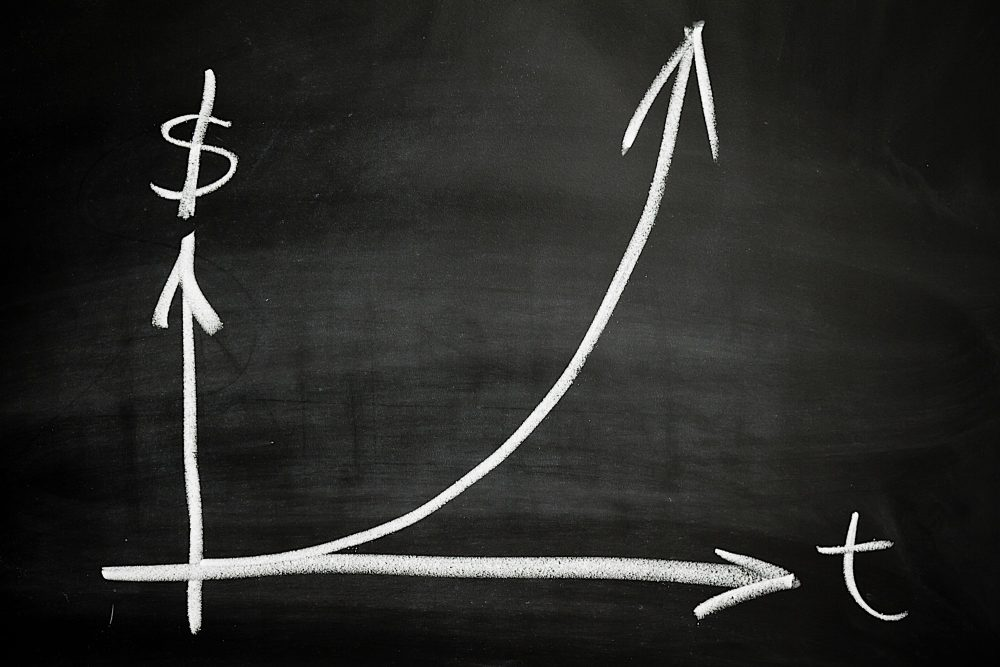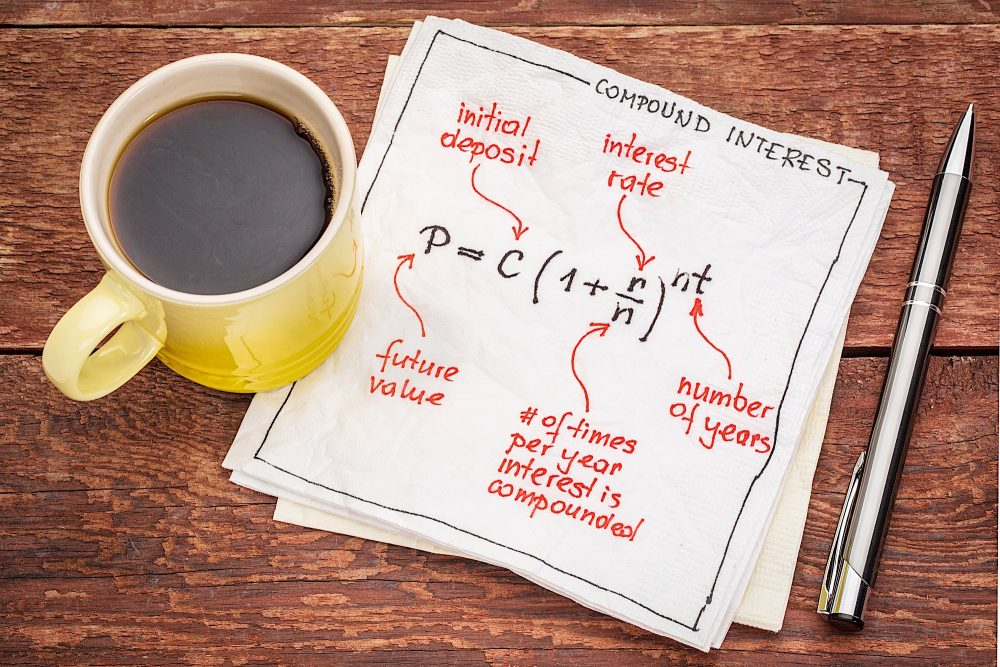# Simple Interest vs. Compound Interest: What’s The Difference?

Key Takeaways

As we all know, there is no such thing as free money. Whether you’re an investor or a household consumer, there will be occasions when you’ll need to borrow funds for investments and purchases. The cost associated with borrowing money is called “interest.” As a borrower, you need to understand the difference between simple interest vs. compound interest, so that you know exactly how much borrowing money will end up costing you. This guide will break down how interest works, as well as the difference between the two types.

## What Is Interest?

Interest is what you’re charged when you borrow money. Most commonly, you are assessed interest charges when taking out a loan from a bank or making purchases on a credit card. It is often expressed as APR, which stands for annual percentage rate. When taking out a loan, two main types of interest can be applied: simple interest or compound interest. Keep reading to find out the difference between simple vs compound interest.

[ What if you could earn money in your sleep? Attend a FREE real estate class offered in your area to discover different passive income strategies in real estate. ]## Simple Interest Vs. Compound Interest

There are two ways a financial institution will calculate interest: simple or compound.

• Simple interest is calculated using only the principal amount of the loan.

• Compound interest is calculated using the principal amount of the loan, plus the interest that has accumulated over previous periods.

The key difference between simple interest vs. compound interest is the amount of interest you’ll end up paying over the life of a loan. You can take its name as a hint; simple interest is easier to understand and to calculate compared to its counterpart. Because you’re only paying interest off of the principal amount of the loan, simple interest is the more affordable option for borrowers.

Compound interest will grow your outstanding balance quickly because your interest accrues its own interest. As a borrower, you’ll want to avoid compound interest as much as possible. For example, credit cards are associated with high annual percentage rates (APR), or interest that is compounded annually. Borrowers often find themselves in hot water when their interest seems to accrue faster than they can pay down the principal.

On the other hand, the fast growth rate associated with compound interest is great news for lenders and investors. It is a powerful tool for building wealth when you can use it to your advantage.

## How To Calculate Simple Interest

Because simple interest is only calculated off of the loan principal, it’s easy to calculate. To calculate simple interest, use the following formula:

Simple Interest = P x r x n

In this case, the variable P is your principal amount, r is your annual interest rate, and n is the term of the loan, expressed in years.

All you have to do is take your loan principal and multiply it by your interest rate, and then multiply the resulting number by the number of years you are taking out the loan for. We’ll show you a couple of examples in the next section.

### Simple Interest Examples

Example 1

Let us say that you are borrowing \$10,000 from Bank A to finance an automobile purchase. You were quoted a simple interest rate of 5 percent, with a loan term of 5 years. The amount of simple interest you will pay, in addition to the principal of \$10,000, will be \$2,500:

\$10,000 x .05 x 5 = \$2,500

Example 2
In this next example, let us say you take out a personal loan from your family toward your first home purchase. They graciously lend you \$40,000 at a 2 percent interest rate. They say you can pay it back slowly over the next 10 years. You will end up paying \$8,000 in interest in this deal.

\$40,000 x .02 x 10 = \$8,000## How Does Compound Interest Work?

Compound interest is a little more intricate than its counterpart, simple interest. Again, compound interest accrues and is added to any interest that was already accumulated in previous periods. In other words, the interest earns its own interest. The formula for compound interest looks like this:

Compound Interest = P[(1+r)^n – 1]

In the formula above, P is the total principal, r is the interest rate, and n is the length of the loan, expressed in years. You calculate compound interest by multiplying the loan principal by one plus the interest rate, which is raised to the number of years of the loan, minus one. You can also put in a different compound period to find out how much compound interest you will have earned at any point in the loan period. It is much easier to explain how this formula works through concrete examples.

### Compound Interest Examples

Example 1
Let’s use the same example from before so that you can see just how much compounded interest will magnify your repayment total. This time, you are taking out a \$10,000 loan from Bank A, but the 5% interest rate is compounded. The loan term is still 5 years. In this case, you’ll end up paying back \$2,762.82 in compounded interest. In the prior example, the simple interest was \$2,500. The compounded rate increases your interest repayment by \$262.82

\$10,000[(1+.05)^5 – 1] = \$10,000 (0.27628)

Example 2
Again, let’s go with Example 2 from above and switch out the simple interest rate with a compounded interest rate. Your family lends you \$40,000, which can be repaid over 10 years. However, this time, they are asking you for 2 percent interest on this loan, to be compounded annually. Instead of an interest repayment of \$8,000, it would be \$8,759.78 when compounded each year. That is roughly \$760 more than before.

\$40,000[(1+.02)^10 – 1] = \$40,000 (0.21899)

## Summary

As you learned from our examples above, it is very important to understand the difference between simple interest vs. compound interest. Compounded interest accrues much more quickly than simple interest, and can result in hundreds of additional dollars in interest repayment. As a borrower, compounded interest can work against you. However, keep in mind that you would enjoy its benefits if you can be on the investing or lending side instead. When finances are involved, it’s always a good idea to analyze the pros and cons and understand what you’re getting yourself into.

Do you have any tricks for committing the simple interest and compound interest formulas to memory? We’d love to hear them! Share in the comments below:

Do you want to be able to retire financially comfortable?

Our new online real estate class can help you learn how to invest in rental properties that can help increase your monthly cash flow. Expert investor Than Merrill shares the core real estate investment principles that work best in today’s real estate market.

Register for our FREE 1-Day Real Estate Webinar and get started educating yourself on how to invest in today’s rental real estate market!

🔒 Your information is secure and never shared. By subscribing, you agree to receive blog updates and relevant offers by email. You can unsubscribe at any time.
Real Estate Investing Strategies

### How To Invest 100k: The 5 Best Ways

By Paul Esajian
Real Estate Investing Strategies

### The Real Estate Investor's Guide To Refinancing Rental Property

By Paul Esajian
Real Estate Investing Strategies

### ARV Meaning & Calculator For Investors

By JD Esajian
Real Estate Investing Strategies

### Real Estate Development Loans You Don't Want To Miss

By Than Merrill
Real Estate Investing Strategies

### Starting And Growing A Real Estate Portfolio The Right Way

By Than Merrill
Real Estate Investing Strategies

By Paul Esajian